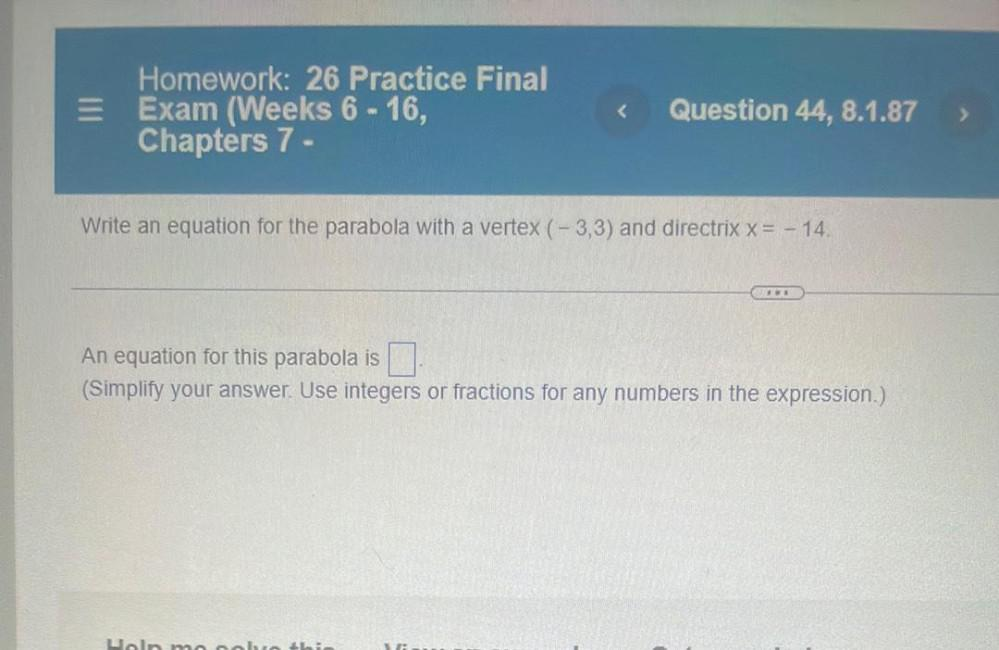Question:

# E Homework: 26 Practice Final Exam (Weeks 6 - 16, Chapters 7 - < Question 44, 8.1.87 > Write an equation for the parabola with aE Homework: 26 Practice Final Exam (Weeks 6 - 16, Chapters 7 - < Question 44, 8.1.87 > Write an equation for the parabola with a vertex (-3,3) and directrix x= - 14. An equation for this parabola is (Simplify your answer. Use integers or fractions for any numbers in the expression.) Uoln moobrobi